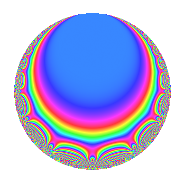# Properties

 Label 189.2.c.bLevel 189 Weight 2 Character orbit 189.c Analytic conductor 1.509 Analytic rank 0 Dimension 4 CM No Inner twists 4

# Learn more about

## Newspace parameters

 Level: $$N$$ = $$189 = 3^{3} \cdot 7$$ Weight: $$k$$ = $$2$$ Character orbit: $$[\chi]$$ = 189.c (of order $$2$$ and degree $$1$$)

## Newform invariants

 Self dual: No Analytic conductor: $$1.5091725982$$ Analytic rank: $$0$$ Dimension: $$4$$ Coefficient field: $$\Q(\sqrt{-3}, \sqrt{-5})$$ Coefficient ring: $$\Z[a_1, \ldots, a_{7}]$$ Coefficient ring index: $$2^{2}$$ Sato-Tate group: $\mathrm{SU}(2)[C_{2}]$

## $q$-expansion

Coefficients of the $$q$$-expansion are expressed in terms of a basis $$1,\beta_1,\beta_2,\beta_3$$ for the coefficient ring described below. We also show the integral $$q$$-expansion of the trace form.

 $$f(q)$$ $$=$$ $$q$$ $$-\beta_{1} q^{2}$$ $$-3 q^{4}$$ $$-\beta_{3} q^{5}$$ $$+ ( -2 + \beta_{2} ) q^{7}$$ $$+ \beta_{1} q^{8}$$ $$+O(q^{10})$$ $$q$$ $$-\beta_{1} q^{2}$$ $$-3 q^{4}$$ $$-\beta_{3} q^{5}$$ $$+ ( -2 + \beta_{2} ) q^{7}$$ $$+ \beta_{1} q^{8}$$ $$+ 5 \beta_{2} q^{10}$$ $$-\beta_{1} q^{11}$$ $$-2 \beta_{2} q^{13}$$ $$+ ( 2 \beta_{1} + \beta_{3} ) q^{14}$$ $$- q^{16}$$ $$-3 \beta_{2} q^{19}$$ $$+ 3 \beta_{3} q^{20}$$ $$-5 q^{22}$$ $$-\beta_{1} q^{23}$$ $$+ 10 q^{25}$$ $$-2 \beta_{3} q^{26}$$ $$+ ( 6 - 3 \beta_{2} ) q^{28}$$ $$+ 2 \beta_{1} q^{29}$$ $$+ \beta_{2} q^{31}$$ $$+ 3 \beta_{1} q^{32}$$ $$+ ( -3 \beta_{1} + 2 \beta_{3} ) q^{35}$$ $$- q^{37}$$ $$-3 \beta_{3} q^{38}$$ $$-5 \beta_{2} q^{40}$$ $$-\beta_{3} q^{41}$$ $$+ 2 q^{43}$$ $$+ 3 \beta_{1} q^{44}$$ $$-5 q^{46}$$ $$-2 \beta_{3} q^{47}$$ $$+ ( 1 - 4 \beta_{2} ) q^{49}$$ $$-10 \beta_{1} q^{50}$$ $$+ 6 \beta_{2} q^{52}$$ $$-4 \beta_{1} q^{53}$$ $$+ 5 \beta_{2} q^{55}$$ $$+ ( -2 \beta_{1} - \beta_{3} ) q^{56}$$ $$+ 10 q^{58}$$ $$+ 2 \beta_{3} q^{59}$$ $$-4 \beta_{2} q^{61}$$ $$+ \beta_{3} q^{62}$$ $$+ 13 q^{64}$$ $$+ 6 \beta_{1} q^{65}$$ $$-10 q^{67}$$ $$+ ( -15 - 10 \beta_{2} ) q^{70}$$ $$+ 5 \beta_{1} q^{71}$$ $$-6 \beta_{2} q^{73}$$ $$+ \beta_{1} q^{74}$$ $$+ 9 \beta_{2} q^{76}$$ $$+ ( 2 \beta_{1} + \beta_{3} ) q^{77}$$ $$+ 2 q^{79}$$ $$+ \beta_{3} q^{80}$$ $$+ 5 \beta_{2} q^{82}$$ $$-2 \beta_{3} q^{83}$$ $$-2 \beta_{1} q^{86}$$ $$+ 5 q^{88}$$ $$+ 3 \beta_{3} q^{89}$$ $$+ ( 6 + 4 \beta_{2} ) q^{91}$$ $$+ 3 \beta_{1} q^{92}$$ $$+ 10 \beta_{2} q^{94}$$ $$+ 9 \beta_{1} q^{95}$$ $$+ 8 \beta_{2} q^{97}$$ $$+ ( -\beta_{1} - 4 \beta_{3} ) q^{98}$$ $$+O(q^{100})$$ $$\operatorname{Tr}(f)(q)$$ $$=$$ $$4q$$ $$\mathstrut -\mathstrut 12q^{4}$$ $$\mathstrut -\mathstrut 8q^{7}$$ $$\mathstrut +\mathstrut O(q^{10})$$ $$4q$$ $$\mathstrut -\mathstrut 12q^{4}$$ $$\mathstrut -\mathstrut 8q^{7}$$ $$\mathstrut -\mathstrut 4q^{16}$$ $$\mathstrut -\mathstrut 20q^{22}$$ $$\mathstrut +\mathstrut 40q^{25}$$ $$\mathstrut +\mathstrut 24q^{28}$$ $$\mathstrut -\mathstrut 4q^{37}$$ $$\mathstrut +\mathstrut 8q^{43}$$ $$\mathstrut -\mathstrut 20q^{46}$$ $$\mathstrut +\mathstrut 4q^{49}$$ $$\mathstrut +\mathstrut 40q^{58}$$ $$\mathstrut +\mathstrut 52q^{64}$$ $$\mathstrut -\mathstrut 40q^{67}$$ $$\mathstrut -\mathstrut 60q^{70}$$ $$\mathstrut +\mathstrut 8q^{79}$$ $$\mathstrut +\mathstrut 20q^{88}$$ $$\mathstrut +\mathstrut 24q^{91}$$ $$\mathstrut +\mathstrut O(q^{100})$$

Basis of coefficient ring in terms of a root $$\nu$$ of $$x^{4}\mathstrut -\mathstrut$$ $$5$$ $$x^{2}\mathstrut +\mathstrut$$ $$25$$:

 $$\beta_{0}$$ $$=$$ $$1$$ $$\beta_{1}$$ $$=$$ $$\nu^{3}$$$$/5$$ $$\beta_{2}$$ $$=$$ $$($$$$2 \nu^{2} - 5$$$$)/5$$ $$\beta_{3}$$ $$=$$ $$($$$$-\nu^{3} + 10 \nu$$$$)/5$$
 $$1$$ $$=$$ $$\beta_0$$ $$\nu$$ $$=$$ $$($$$$\beta_{3}\mathstrut +\mathstrut$$ $$\beta_{1}$$$$)/2$$ $$\nu^{2}$$ $$=$$ $$($$$$5$$ $$\beta_{2}\mathstrut +\mathstrut$$ $$5$$$$)/2$$ $$\nu^{3}$$ $$=$$ $$5$$ $$\beta_{1}$$

## Character Values

We give the values of $$\chi$$ on generators for $$\left(\mathbb{Z}/189\mathbb{Z}\right)^\times$$.

 $$n$$ $$29$$ $$136$$ $$\chi(n)$$ $$-1$$ $$-1$$

## Embeddings

For each embedding $$\iota_m$$ of the coefficient field, the values $$\iota_m(a_n)$$ are shown below.

For more information on an embedded modular form you can click on its label.

Label $$\iota_m(\nu)$$ $$a_{2}$$ $$a_{3}$$ $$a_{4}$$ $$a_{5}$$ $$a_{6}$$ $$a_{7}$$ $$a_{8}$$ $$a_{9}$$ $$a_{10}$$
188.1
 1.93649 + 1.11803i −1.93649 + 1.11803i 1.93649 − 1.11803i −1.93649 − 1.11803i
2.23607i 0 −3.00000 −3.87298 0 −2.00000 + 1.73205i 2.23607i 0 8.66025i
188.2 2.23607i 0 −3.00000 3.87298 0 −2.00000 1.73205i 2.23607i 0 8.66025i
188.3 2.23607i 0 −3.00000 −3.87298 0 −2.00000 1.73205i 2.23607i 0 8.66025i
188.4 2.23607i 0 −3.00000 3.87298 0 −2.00000 + 1.73205i 2.23607i 0 8.66025i
 $$n$$: e.g. 2-40 or 990-1000 Significant digits: Format: Complex embeddings Normalized embeddings Satake parameters Satake angles

## Inner twists

Char. orbit Parity Mult. Self Twist Proved
1.a Even 1 trivial yes
3.b Odd 1 yes
7.b Odd 1 yes
21.c Even 1 yes

## Hecke kernels

This newform can be constructed as the kernel of the linear operator $$T_{2}^{2}$$ $$\mathstrut +\mathstrut 5$$ acting on $$S_{2}^{\mathrm{new}}(189, [\chi])$$.